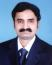# Calculate Estimated cost while SV and CV are given

The schedule variance for a project is 20%.The Budget for the project is \$480,000. What is the estimated cost on project completion if the EV and AC of the project currently is \$48,000 and \$60,000 respectively and if the current cost variance and schedule variance is expected to continue in future?

 a. \$500,000. b. \$600,000. c. \$527,500. d. \$620,000.

Kindly helpKey Point: Current Vairance is expected to continue in future. Then here is the formula for EAC:

EAC = BAC/CPI

First Calculate CPI = 48,000/60,000 = 0.8

You know the BAC = 480,000

Then apply the formula: EAC = 480,000/0.8 = 600,000.